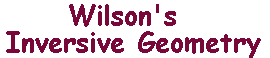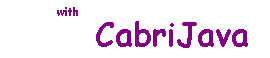The inverses of (extended) lines and circles

To make the statement of the following theorem as simple as possible,
we use the notions of the extended lines.

 The Inversion Theorem Let C be a circle with centre O. For any object X, let X' denote the inverse of X with respect to C. If L is an extended line through O, then L' = L If L is an extended line not through O, then L' is a circle through O. If D is a circle through O, then D' is an extended line through O If D is a circle not through O, then D' is a circle not through O Proof of the Inversion Theorem There is a neater (algebraic) proof on the Algebraic Inversion Theorem page

CabriJava illustrations of cases 2 and 4

 This shows the case where we are inverting an extended line L in the Circle C. If you move Q (on L), you will see that the image always lies on the circle L'. This shows the case where we are invering a circle D in the circle C. If you move P on D, you will see that P' always lies on the circle D'.

The importance of this result is that it shows that, if J is either a circle or an extended line,
then the inverse will be a circle or extended line.

Thus, the Theorem says that inversion maps i-lines to i-lines!

These i-lines play much the same role in inversive geometry thet lines do in euclidean geometry.

We now look at the the effect of inversion on angles between i-lines.